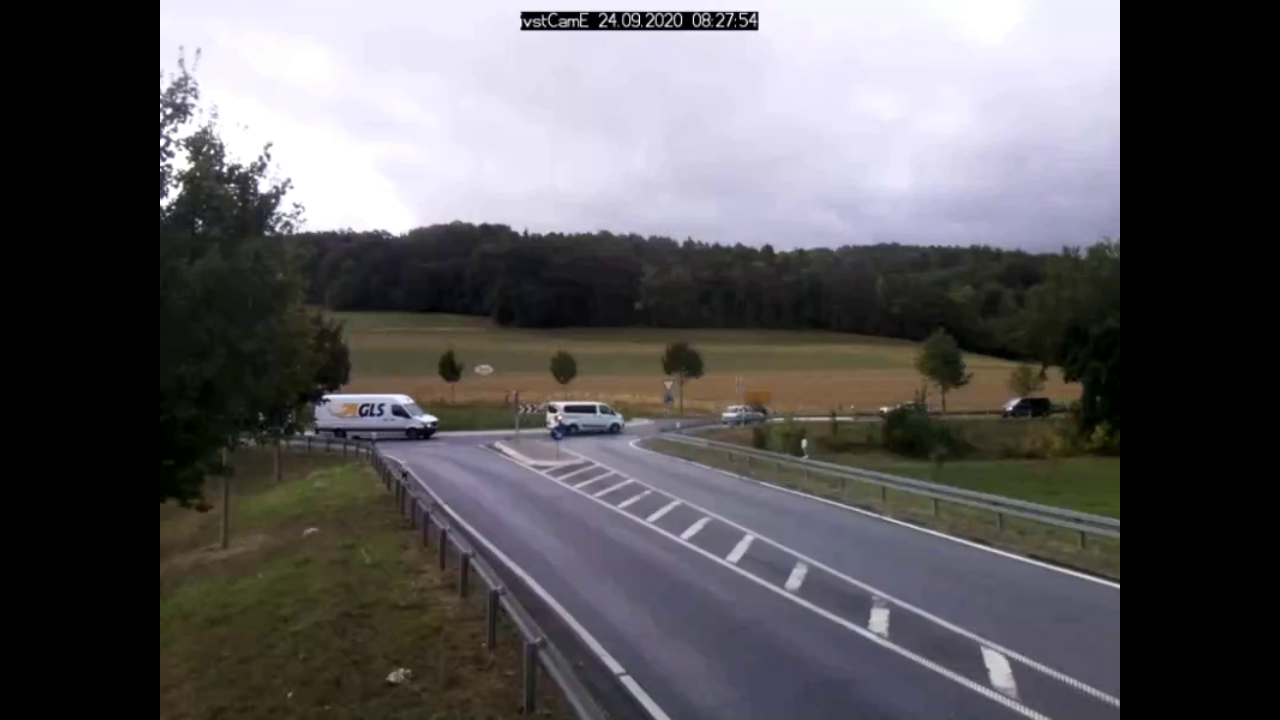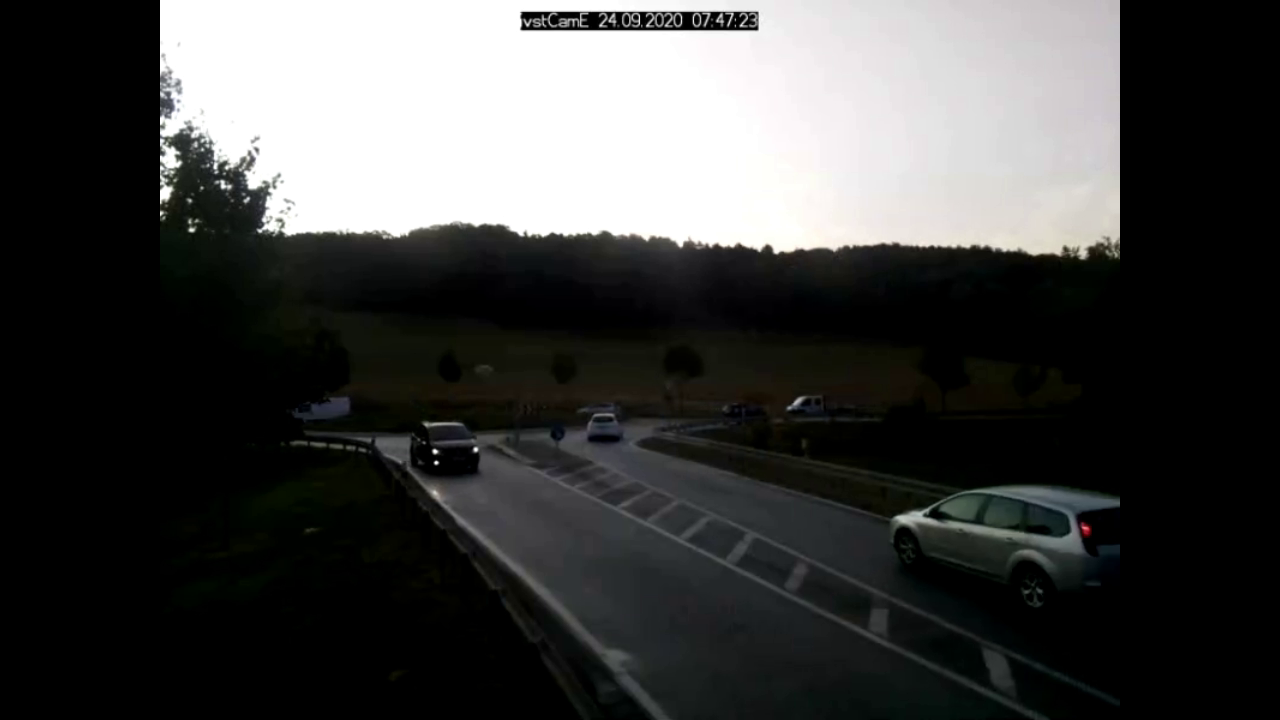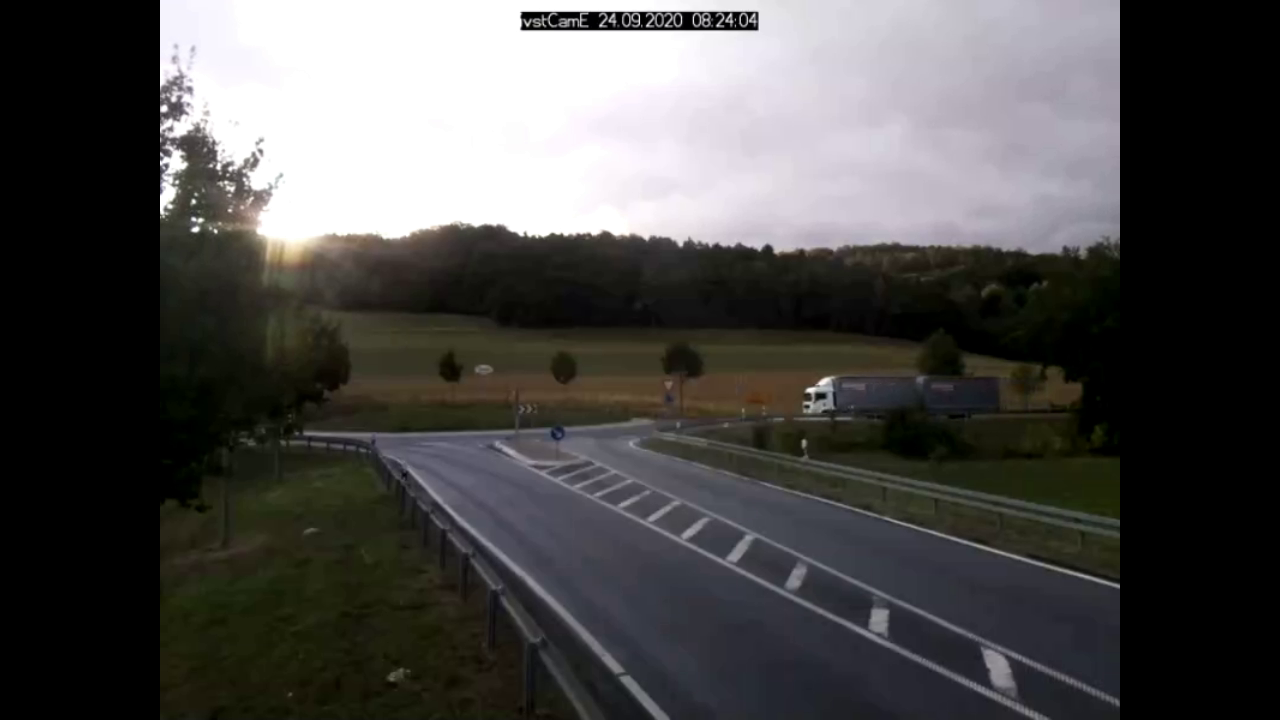# Peak Signal-to-Noise-Ratio

Full-Reference Quality Metrics

## Description

The Peak Signal-to-Noise-Ratio (PSNR) expands the Mean Squared Error (MSE) by scaling the average squared difference between actual and ideal pixel values to the pixel range. It therefore compares an ideal reference image with an actual image and uses the maximum pixel value for scalation.

## Interpretation

When an image has no pixel differences (no errors), the PSNR equals zero. As image differences increase, its value increases depending on the maximum pixel value.

## Limits

PSNR is simple to calculate but might not align well with the human perception of quality.

Peak Signal-to-Noise Ratio (PSNR) avoids the problem of the MSE, which strongly depends on the image intensity, by scaling the MSE according to the pixel range.

Only images showing the same scene should be compared.

## Example

An image from a traffic surveillance camera in Germany is used to show the PSNR results.

Reference ImagePSNR of 2075.3PSNR of 917.8## Tools and Libraries

### Python

In Python the package sewar contains multiple image quality metrics. One of them is the PSNR.

Install package:

```pip install sewar
```

Calculate PSNR:

```from sewar.full_ref import psnr
import cv2

score_dark = psnr(img_ref,img_dark)
print("Score of dark image", score_dark)

score_sun = psnr(img_ref,img_sun)
print("Score of dark image", score_sun)
```

### MATLAB

Within the MATLAB Image Processing Toolbox a function to calculate the PSNR exists:

```ref = imread('Reference_Image.png');

PSNR_dark = psnr(dark, ref);
fprintf('The PSNR for the dark image is %0.4f\n', PSNR_dark);

PSNR_sun = psnr(sun, ref);
fprintf('The PSNR for the sun image is %0.4f\n', PSNR_sun);
```

A detailed description can be found at the Mathworks Website. For an RGB image the PSNR for each channel is calculated and the average of all channel PSNRs is the PSNR of the image. It is also possible to only calculate the PSNR for one channel:

```ref = imread('Reference_Image.png');

PSNR_R = psnr(dark(:,:,1), ref(:,:,1));
PSNR_G = psnr(dark(:,:,2), ref(:,:,2));
PSNR_B = psnr(dark(:,:,3), ref(:,:,3));
fprintf('\nThe PSNR for R-channel %0.4f\n', PSNR_R);
fprintf('The PSNR for G-channel %0.4f\n', PSNR_G);
fprintf('The PSNR for B-channel %0.4f\n', PSNR_B);
```

If access to the MATLAB Image Processing Toolbox is denied, one can program the PSNR by their own:

```num_pixel = size(ref,1)*size(ref,2)*size(ref,3);
MSE = sum((double(ref) - double(dark)).^2,'all') / num_pixel;
max_pixel = double(max(dark,[],'all'));
PSNR = -10*log10(MSE/(max_pixel*max_pixel));
fprintf('The PSNR for the dark image is %0.4f\n', PSNR);
```

### C++

OpenCV contains a class for calculating the PSNR. A detailed description can be found in the OpenCV Docs:

```#include <opencv2/quality/qualitypsnr.hpp>
#include <iostream>

int main()
{
std::string image_path = samples::findFile("Reference_Image.png");
std::string image_path = samples::findFile("Image_Dark.png");